## Estimate Regularized ARX Model Using System Identification App

This example shows how to estimate regularized ARX models using automatically generated regularization constants in the System Identification app.

### Open a saved System Identification App session.

```filename = fullfile(matlabroot,'help','toolbox',... 'ident','examples','ex_arxregul.sid'); systemIdentification(filename)```

The session imports the following data and model into the System Identification app:

• Estimation data `eData`

The data is collected by simulating a system with the following known transfer function:

`$G\left(z\right)=\frac{0.02008+0.04017{z}^{-1}+0.02008{z}^{-2}}{1-1.56{z}^{-1}+0.6414{z}^{-2}}$`
• Transfer function model `trueSys`

`trueSys` is the transfer function model used to generate the estimation data `eData` described previously. You also use the impulse response of this model later to compare the impulse responses of estimated ARX models.### Estimate a 50th-order ARX model.

1. In the System Identification app, select Estimate > Polynomial Models to open the Polynomial Models dialog box.

2. Verify that ARX is selected in the Structure list.

3. In the Orders field, specify [0 50 0] as the ARX model order and delay.4. Click to estimate the model.

A model `arx0500` is added to the System Identification app.

### Estimate a 50th-order regularized ARX model.

1. In the Polynomial Models dialog box, click .

2. In the Regularization Options dialog box, select `TC` from the Regularization Kernel drop-down list.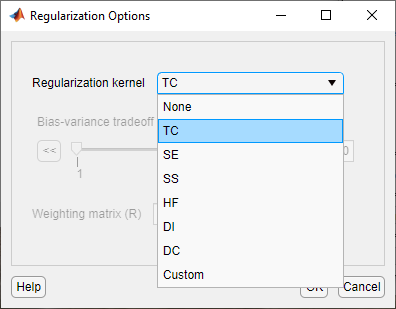Specifying this option automatically determines regularization constants using the `TC` regularization kernel. To learn more, see the `arxRegul` reference page.

Click to close the dialog box.

3. In the Name field in the Polynomial Models dialog box, type `arx0500reg`.

4. Click .

A model `arx0500reg` is added to the System Identification app.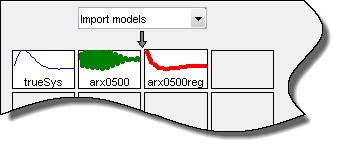### Compare the unregularized and regularized model outputs to estimation data.

Select the Model output check box in the System Identification app.

The Measured and simulated model output plot shows that both the models have an 84% fit with the data.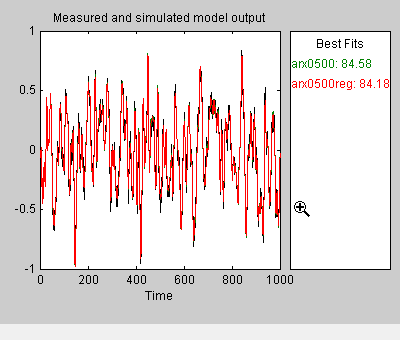### Determine if regularization leads to parameter values with less variance.

Because the model fit to the estimation data is similar with and without using regularization, compare the impulse response of the ARX models with the impulse responses of `trueSys`, the system used to collect the estimation data.

1. Click the `trueSys` icon in the model board of the System Identification app.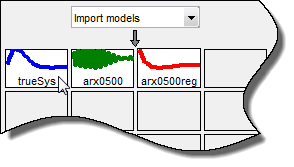2. Select the Transient resp check box to open the Transient Response plot window.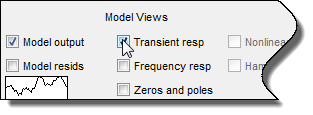By default, the plot shows the step response.

3. In the Transient response plot window, select Options > Impulse response to change to plot to display the impulse response.

4. Select Options > Show 99% confidence intervals to plot the confidence intervals.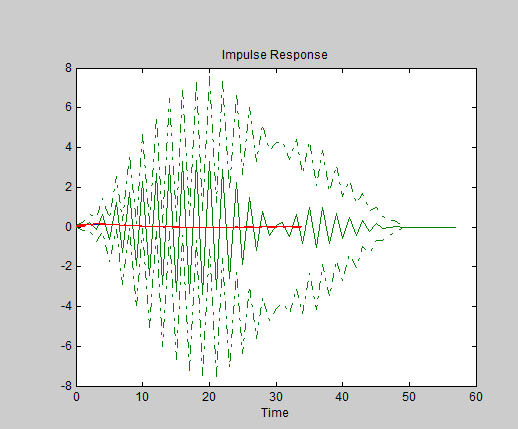The plot shows that the impulse response of the unregularized model `arx0500` is far off from the true system and has large uncertainties.

To get a closer look at the model fits to the data and the variances, magnify a portion of the plot.The fit of the regularized ARX model `arx0500reg` closely matches the impulse response of the true system and the variance is greatly reduced as compared to the unregularized model.

## Related Examples